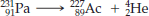×
Get Full Access to Introductory Chemistry - 5 Edition - Chapter 17 - Problem 20q
Get Full Access to Introductory Chemistry - 5 Edition - Chapter 17 - Problem 20q

×

# Identify the parent nuclides and daughter nuclides in the nuclear equation. Which kindISBN: 9780321910295 34

## Solution for problem 20Q Chapter 17

Introductory Chemistry | 5th Edition

• Textbook Solutions
• 2901 Step-by-step solutions solved by professors and subject experts
• Get 24/7 help from StudySoup virtual teaching assistantsIntroductory Chemistry | 5th Edition

4 5 1 319 Reviews
10
5
Problem 20Q

Problem 20Q

Identify the parent nuclides and daughter nuclides in the nuclear equation. Which kind of radioactive decay is involved?Step-by-Step Solution:

Solution 20Q :

Step 1:

Here, we have to identify the parent nuclides and daughter nuclides in the nuclear equation and state the kind of radioactive decay is involved.In the chemical equation, the original atom is called the parent nuclide and the products are called as the daughter nuclides. When an element emits an alpha particle, the number of protons in its nucleus changes, transforming it into a different element.

Step 2 of 3

Step 3 of 3

##### ISBN: 9780321910295

Unlock Textbook Solution